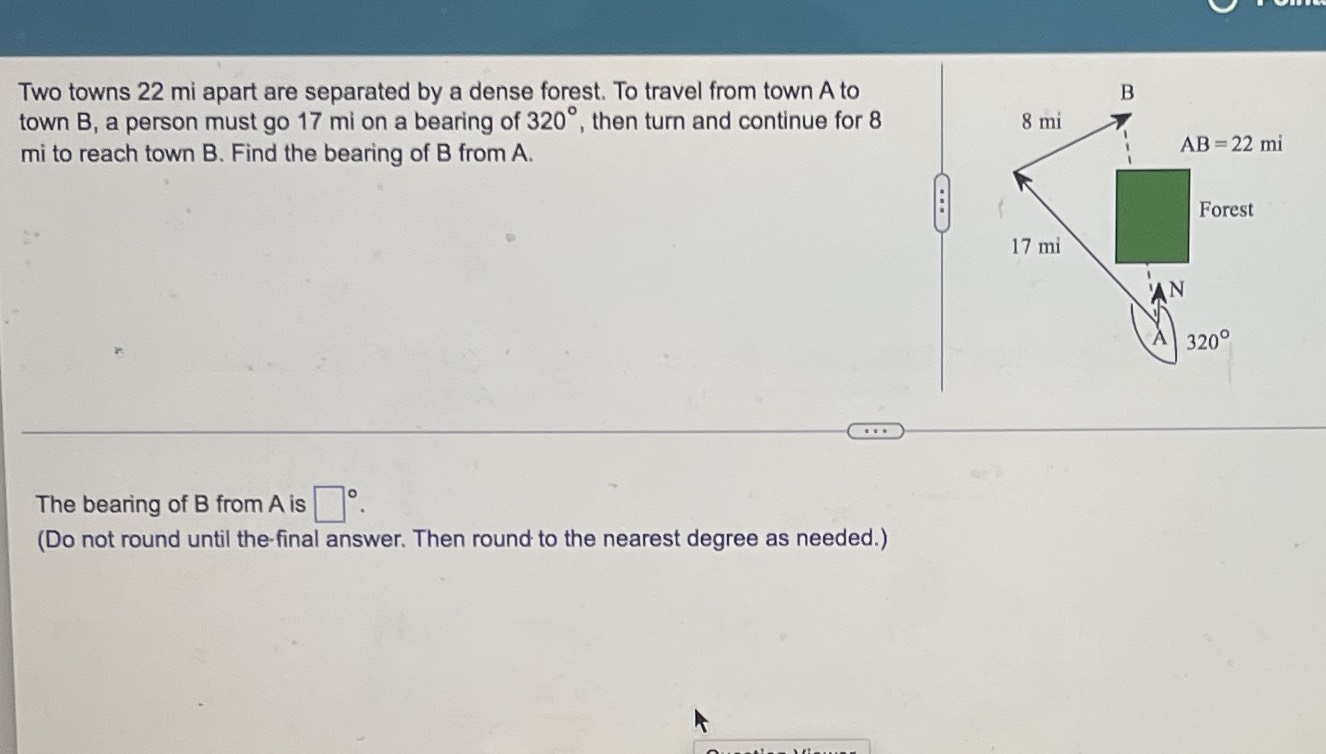### Still have math questions?Two towns $$22$$ mi apart are separated by a dense forest. To travel from town $$A$$ to town $$B$$ , a person must go $$17$$ mi on a bearing of $$320 ^ { \circ }$$ , then turn and continue for $$8$$ mi to reach town $$B$$ . Find the bearing of $$B$$ from $$A$$ . The bearing of $$B$$ from $$A$$ is $$\square ^ { \circ }$$ . (Do not round until the-final answer. Then round to the nearest degree as needed.)